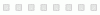## Determining the Thermal Conductivity of Thin Ceramics

During the design phase of a power module, engineers select the components, materials and manufacturing technologies to fulfill the requirements regarding performance, reliability and costs set by their customers. Over-engineering can be desirable when safety, reliability and performance are critical. However, the performance to price ratio (or watt per dollar) increases with every new module generation. Consequently, engineers have to simulate their modules to operate safely and within their constrained limits. Particularly, the thermal conductivity of the raw material ceramic and its temperature dependency are important parameters that need to be taken into account for a robust and cost-effective design. Let us take a deep dive to understand how the thermal conductivity is determined and how accurate the measurement methods are.

Thermal conductivity describes the transport of energy – in the form of heat – through a body of mass, as the result of a temperature gradient. It is an intrinsic property of a homogeneous material which describes the material’s ability to conduct heat. This property is independent of material´s size, shape or orientation. It can be calculated using the following equation: λ (T) = ρ (T) * cp(T) * a(T)

Where:

λ (T): thermal conductivity [W/m*K]

ρ(T): density [g/cm3]

cp(T): specific heat capacity [J/g*K]

a(T): thermal diffusivity [mm2/s]

Density describes the relation between mass and volume of a material. Specific heat capacity is the amount of heat that is necessary to increase the temperature of one gram of material by one Kelvin. Thermal diffusivity indicates how quickly a material reacts to a temperature change.

Thermal diffusivity is often measured with the laser flash method (LFA). It involves heating a sample with a short energy pulse at one end and analyzing the temperature change at the other end. This requires precise measurement of the sample thickness with a micrometer. The measurement probe tip should be sharp enough to exclude any error due to the bending of the samples. Moreover, thin samples need to be coated with graphite to ensure a good coupling of the laser source to the sample. Furthermore, a dense and very homogeneous graphite layer is required to avoid measurement errors. In this regard, hand spraying of graphite is prone to error and an automated spraying system should be preferred. Finally, the measurement result also depends on the pulse width of the laser. In the end, a measurement uncertainty in the range of three percent is typical for thermal diffusivity.

The specific heat capacity of a material is typically determined by measuring the heat capacity of a sample material and dividing it by the samples mass. Differential Scanning Calorimetry (DSC) is the most-employed thermal analysis method for estimating the heat capacity of a material. DSC measurements require that samples are enclosed in a sample pan in order to avoid the direct contact between sample and furnace and/or sensor. Special care is required for the selection and handling of the pans because this can have a significant impact on the reproducibility of the measurement results. For this measurement method the uncertainty range is typically five percent.

The density as a function of temperature ρ(T) is determined by measurement of the thermal expansion coefficient (CTE) and the density at room temperature ρ0(RT). The density at room temperature can be measured by the buoyancy method. It involves weighing the sample twice in two different mediums, typically in air and liquid. With known densities of the air and the applied liquid, the density of the sample can be calculated. This sounds easy but measuring the density of thin ceramics require special care. The thermal expansion coefficient α(T) is measured by thermo-mechanical analysis (TMA). Thin ceramics are usually stacked to achieve the required sample thickness of 3mm. This results in an inhomogeneous sample with problems of deformation under temperature. Finally, α(T) is only determined in z-direction. Again, an uncertainty in the range of five percent is typical for this measurement method.

Ultimately thermal conductivity is calculated based on the measurement results and their uncertainties for each of the three variables. This results in an even higher uncertainty, typically in the range of 20%. Nevertheless, over the years, we have measured and calculated the thermal conductivity from our ceramic materials and from various suppliers. The thermal conductivity depends on temperature, which decreases as temperature increases. These values are particularly useful to simulate the thermal performance of a given power module and are available on request.

To conclude, determining thermal conductivity is a very challenging task. It requires knowledge of the sample including the sample’s geometry, size and preparation method. Understanding the fundamentals and procedures of the testing technique is another prerequisite. Finally, it is important to be aware of the potential error sources which might affect the results.